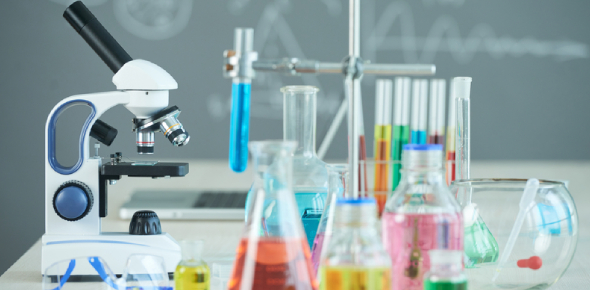# Chemistry MCQ Trivia Exam! Quiz

20 Questions | Total Attempts: 34Settings• 1.
The particles that are located in the nucleus of an atom are:
• A.

Protons and neutrons

• B.

Protons and electrons

• C.

Neutrons and electrons

• D.

Only protons

• 2.
The particles that are negatively charged are:
• A.

Protons

• B.

Neutrons

• C.

Electrons

• D.

Only valence electrons

• 3.
What is the electron configuration for Nitrogen?
• A.

2, 5

• B.

2, 5, 5

• C.

2,8, 1

• D.

1

• 4.
What are some of the elements that are in the noble gases group?
• A.

Hydrogen and helium

• B.

Helium, neon and argon

• C.

Lithium, sodium and potassium

• D.

Beryllium, magnesium and calcium

• 5.
The group containing the elements lithium, sodium, potassium, rubidium, caesium and francium is:
• A.

Non-metals

• B.

Noble gases

• C.

Alkaline earth metals

• D.

Alkali metals

• 6.
Fluorine, chlorine and bromine are part of which group?
• A.

Noble gases

• B.

Halogens

• C.

Alkaline earth metals

• D.

Other non-metals

• 7.
The electron configuration for Helium is:
• A.

1

• B.

2

• C.

2, 4

• D.

2, 8, 1

• 8.
Why are noble gases inert?
• A.

Because they are stable

• B.

Because their valence electron shell is full

• C.

Because they are unstable

• D.

A and b only

• E.

B and c only

• 9.
What are isotopes?
• A.

The same element but with differing mass

• B.

Different elements of the same mass

• C.

The same element with a different charge

• D.

Reactive elements

• 10.
Which of the following elements is a metal?
• A.

Oxygen

• B.

Selenium

• C.

Calcium

• D.

• 11.
Which of the following is a non-metal?
• A.

Argon

• B.

Potassium

• C.

Calcium

• D.

Barium

• 12.
A           E     Looking at the following representation, 'A' denotes: Z
• A.

The atomic mass

• B.

The atomic number

• C.

The element symbol

• D.

The number of protons

• 13.
To determine the number of neutrons in an atom, the equation used is:
• A.

It is found on the periodic table

• B.

It is the same as the number of protons

• C.

Atomic number - atomic mass = number of neutrons

• D.

Atomic mass - atomic number = number of neutrons

• 14.
The symbol for mercury is:
• A.

Mg

• B.

Hg

• C.

Mc

• D.

Ag

• 15.
What is the symbol for Manganese?
• A.

Mg

• B.

Mn

• C.

Mo

• D.

Ag

• 16.
The formulae NaCl represents which compound?
• A.

Sodium chloride

• B.

Water

• C.

Sulphur trioxide

• D.

Ammonia

• 17.
What is a valence shell?
• A.

The outermost electron shell

• B.

The innermost electron shell

• C.

Another term for electron shell

• 18.
Methane has a formulae of:
• A.

CH4

• B.

CuCO3

• C.

H2SO4

• D.

NaCl

• 19.
Lithium oxide has a formulae of:
• A.

CH4

• B.

Li2O

• C.

SiO2

• D.

CaS

• 20.
Calcium has an electron configuration of:
• A.

2, 8, 8, 5

• B.

2, 8, 1

• C.

2, 8, 8, 2

• D.

2, 8, 5

Related TopicsBack to top